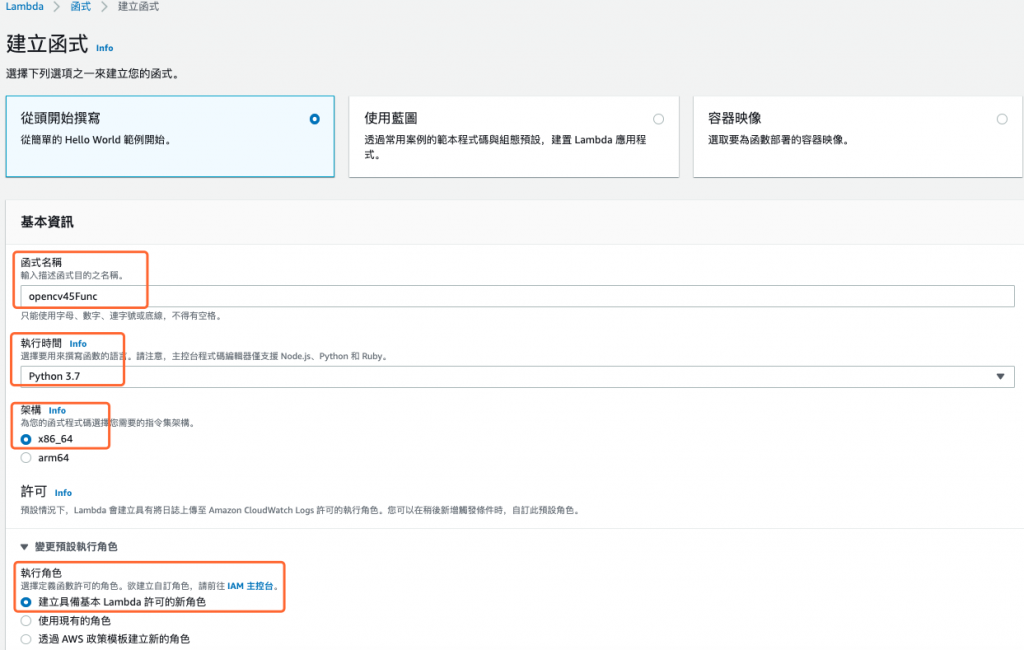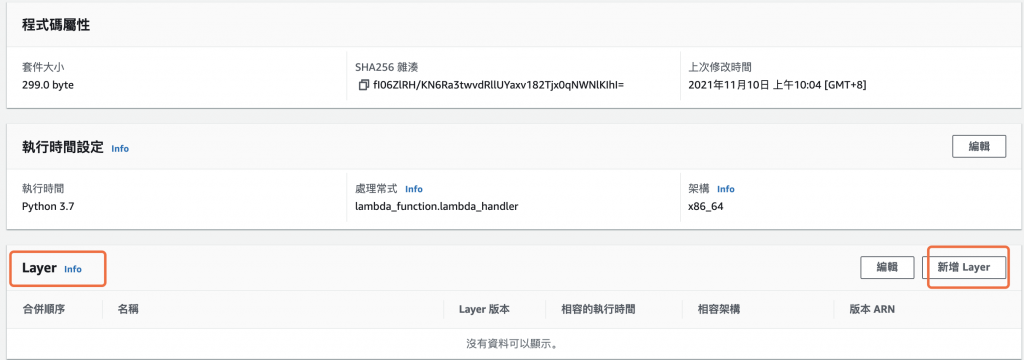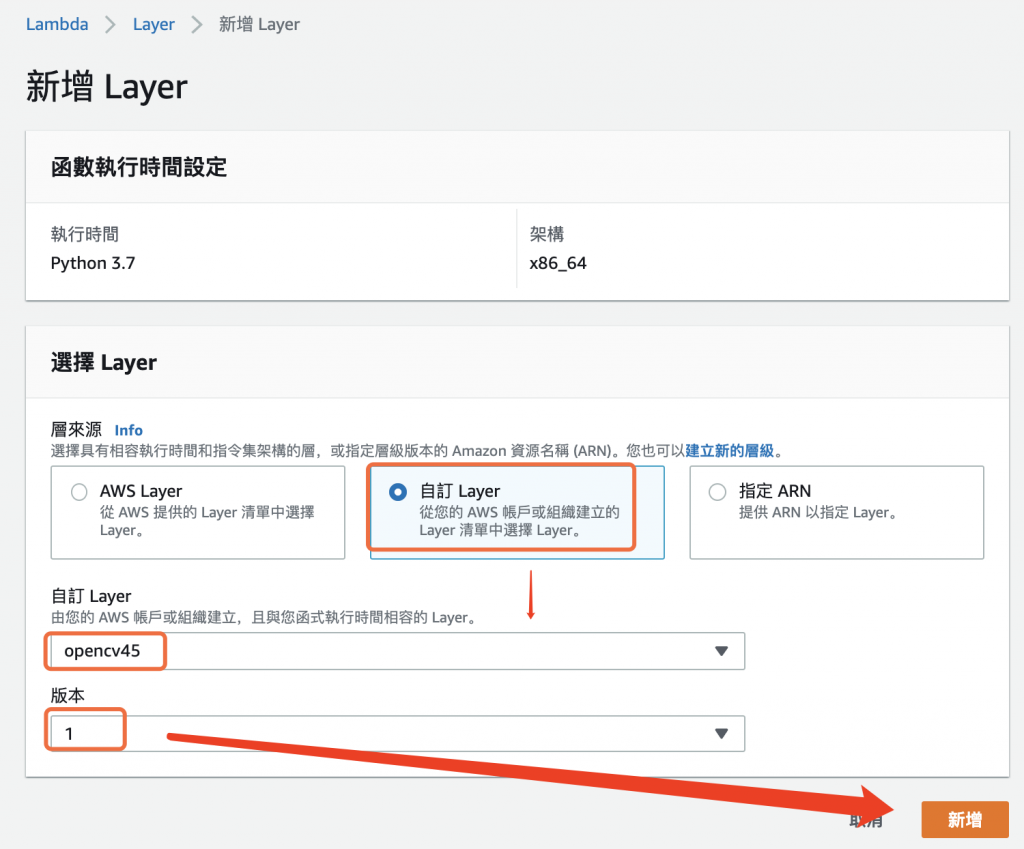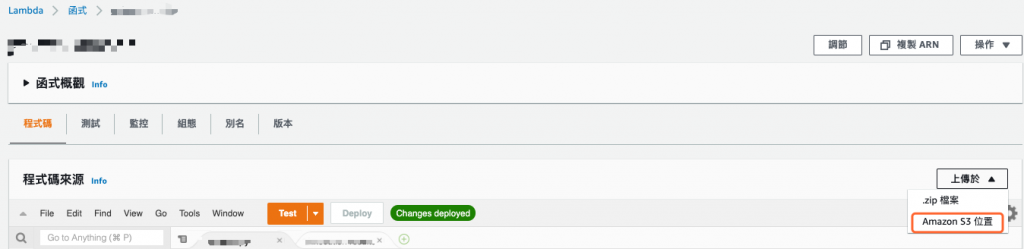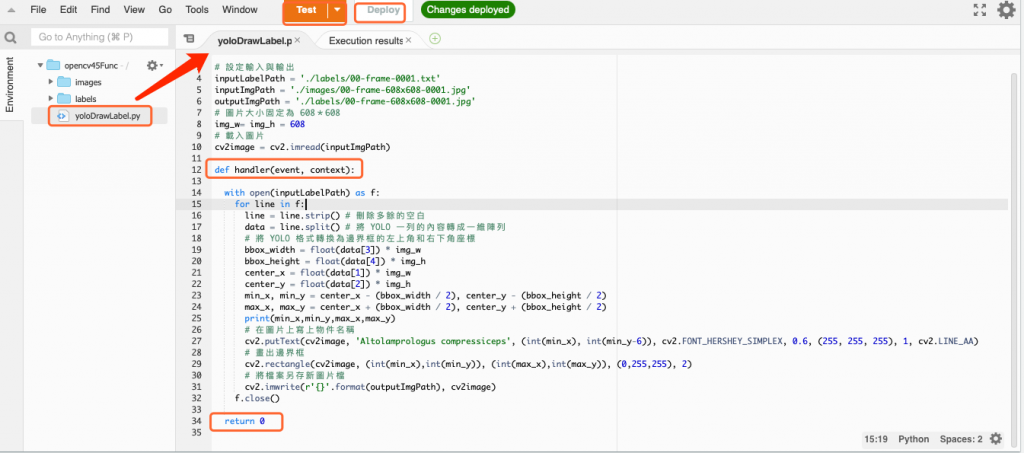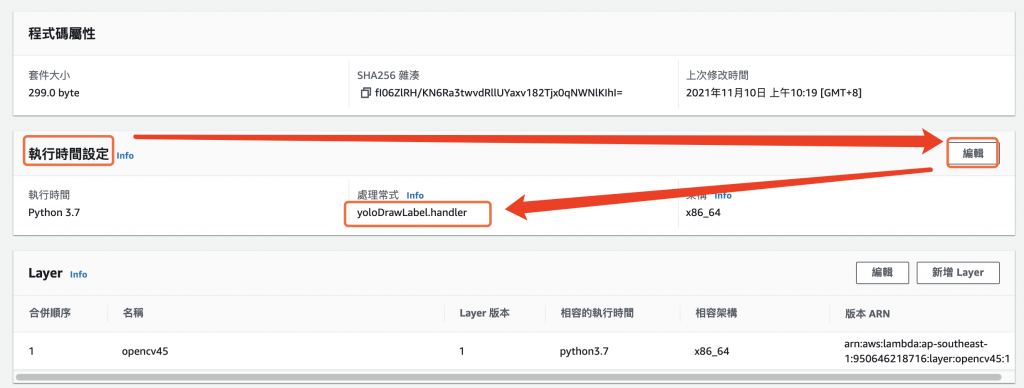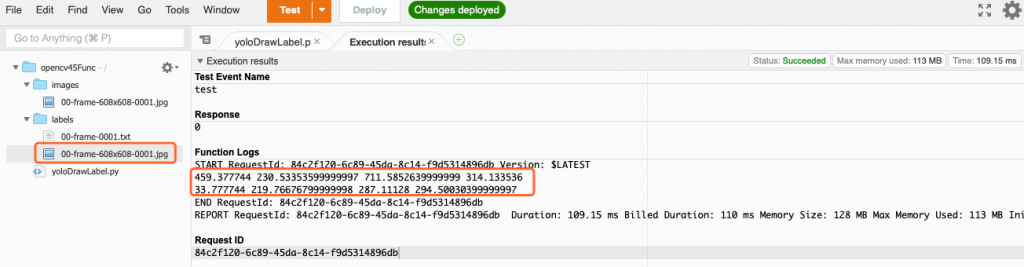0
AI & Data

Day 37 - 在 AWS Lambda 建立 OpenCV Layer

1. 打包 Python 的 OpenCV 函式庫
2. 在 AWS Lambda 建立 OpenCV 層
3. 打包程式以及圖片上傳到 S3
4. 在 AWS Lambda 執行

• 創建一個新的 Python 虛擬環境
• 檢查 Python 版本
• 更新 pip
• 安裝 opencv
• 將安裝好的套件壓縮到 python 這個目錄下
• 檢視壓縮內容
python3 -m venv opencv
cd opencv
. bin/activate
python -V
pip install pip -U
pip install opencv-python
cd lib/python3.7/
ln -s site-packages/ python
zip -r opencv37-45.zip python/
unzip -l opencv37-45.zip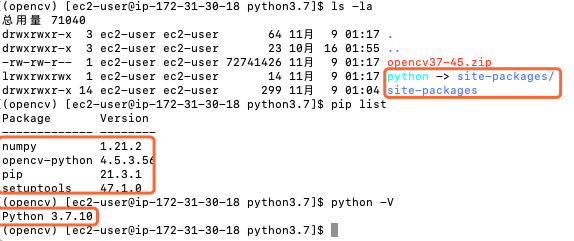aws s3 cp opencv37-45.zip s3://[S3存儲桶的名稱]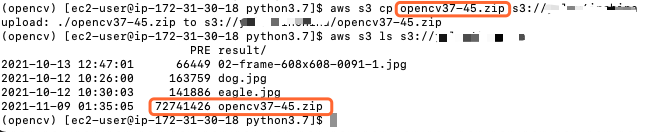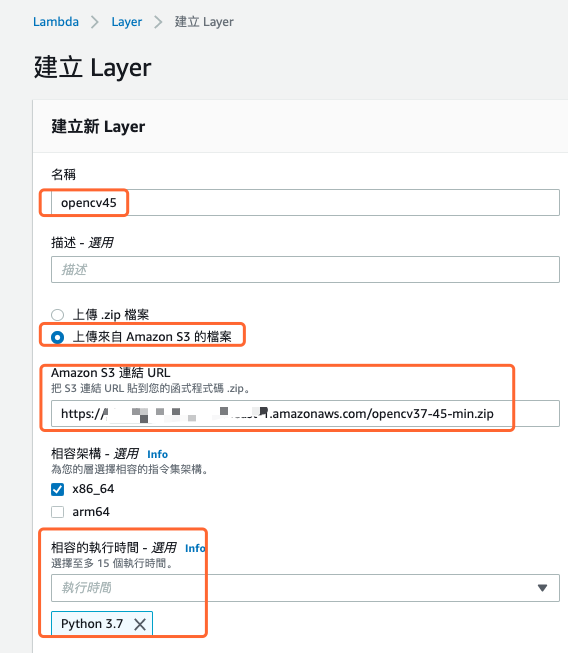# 建立 lambda 專屬目錄，目錄名稱沒有強制要求
mkdir lambda
cd lambda
# 產生使用 opencv 的程式碼
vi yoloDrawLabel.py
mkdir labels images
# 上傳標籤所需要的圖片與標籤檔
aws s3 cp s3://[S3存儲桶的名稱]/00-frame-608x608-0001.jpg ./images
aws s3 cp s3://[S3存儲桶的名稱]/00-frame-0001.txt ./labels
# 執行程式，確保可以在虛擬環境中執行
python yoloDrawLabel.py
# 將所需要檔案壓縮並上傳到 S3
zip -r lambda.zip .
aws s3 cp lambda.zip s3://[S3存儲桶的名稱]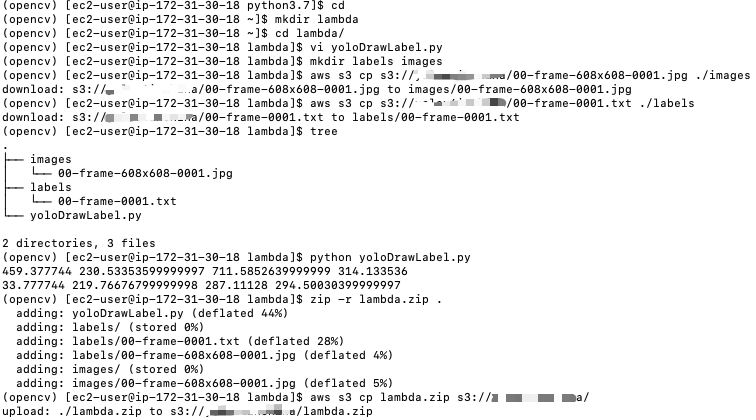./yoloDrawLabel.py

import cv2

# 設定輸入與輸出
inputLabelPath = './labels/00-frame-0001.txt'
inputImgPath = './images/00-frame-608x608-0001.jpg'
outputImgPath = './labels/00-frame-608x608-0001.jpg'
# 圖片大小固定為 608＊608
img_w= img_h = 608
# 載入圖片

with open(inputLabelPath) as f:
for line in f:
line = line.strip() # 刪除多餘的空白
data = line.split() # 將 YOLO 一列的內容轉成一維陣列
# 將 YOLO 格式轉換為邊界框的左上角和右下角座標
bbox_width = float(data) * img_w
bbox_height = float(data) * img_h
center_x = float(data) * img_w
center_y = float(data) * img_h
min_x, min_y = center_x - (bbox_width / 2), center_y - (bbox_height / 2)
max_x, max_y = center_x + (bbox_width / 2), center_y + (bbox_height / 2)
print(min_x,min_y,max_x,max_y)
# 在圖片上寫上物件名稱
cv2.putText(cv2image, 'Altolamprologus compressiceps', (int(min_x), int(min_y-6)), cv2.FONT_HERSHEY_SIMPLEX, 0.6, (255, 255, 255), 1, cv2.LINE_AA)
# 畫出邊界框
cv2.rectangle(cv2image, (int(min_x),int(min_y)), (int(max_x),int(max_y)), (0,255,255), 2)
# 將檔案另存新圖片檔
cv2.imwrite(r'{}'.format(outputImgPath), cv2image)
f.close()

• 選擇下列選項之一來建立您的函式： 從頭開始撰寫
• 函式名稱： opencv45Func
• 執行時間： Python 3.7 （注意：這個選項不能選其他的 Python 版本，會出現錯誤的）
• 架構： x86_64
• 執行角色： 建立具備基本 Lambda 許可的新角色